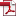• KSII Transactions on Internet and Information Systems
Monthly Online Journal (eISSN: 1976-7277)

# Resource Allocation in Multi-User MIMO-OFDM Systems with Double-objective Optimization

Vol. 12, No. 5, May 30, 201810.3837/tiis.2018.05.009, Download Paper (Free):#### Abstract

A resource allocation algorithm is proposed in this paper to simultaneously minimize the total system power consumption and maximize the system throughput for the downlink of multi-user multiple input multiple output-orthogonal frequency division multiplexing (MIMO-OFDM) systems. With the Lagrange dual decomposition method, we transform the original problem to its convex dual problem and prove that the duality gap between the two problems is zero, which means the optimal solution of the original problem can be obtained by solving its dual problem. Then, we use convex optimization method to solve the dual problem and utilize bisection method to obtain the optimal dual variable. The numerical results show that the proposed algorithm is superior to traditional single-objective optimization method in both the system throughput and the system energy consumption.